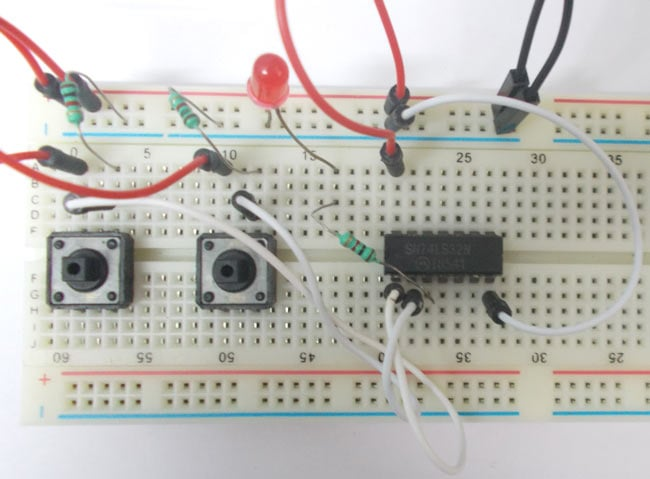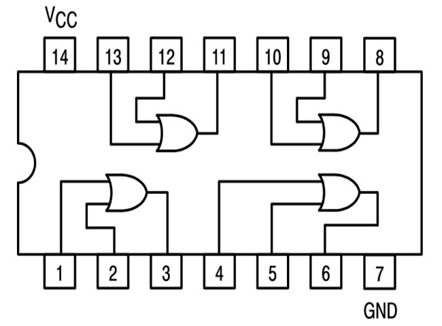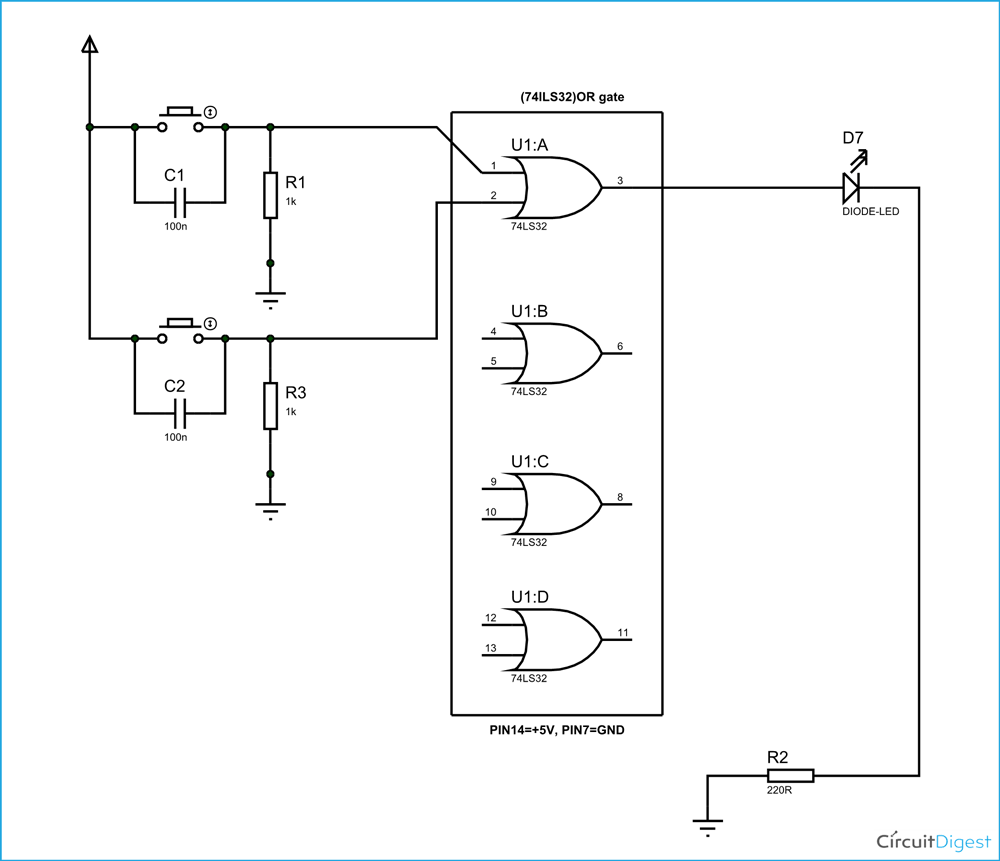# OR Gate

Published  September 5, 2015   0OR gate is a digital logic gate, designed for arithmetic and logical operations. This gate is mainly used in applications where there is a need for mathematical calculations. Here we are going to use 74LS32 chip for demonstration, this chip has 4 OR gates in it. These four gate are connected internally as shown in below figure.These gates have limitations for working voltage and input logic frequency. When these limitations are not considered the chip may damage permanently, so one should pay attention while selecting the  logic gates.

### Components

• Power supply (5v)
• 1K resistor (2 pieces)
• 220Ω resistor
• 1 LEDS
• Buttons (2 pieces)
• 100nF capacitor (2 pieces)

### Circuit Diagram and Working

The truth table of OR gate is show in figure.

 A B X 0 0 0 0 1 1 1 0 1 1 1 1

As in truth table the output of a OR gate should be LOW only if both the gate inputs are LOW.  In any other case the output should be HIGH. So if any one or both inputs are HIGH,the output of OR gate will be HIGH.

In this OR gate circuit we are going to pull down both input of a gate to ground through a 1KΩ resistor.  And then the inputs are connected to power through a button. So when the button is pressed the corresponding pin of gate INPUT goes high. So with two buttons we can realize the truth table of OR gate. When one of button is pressed one input of gate will be high and other will be low at this time the output should be HIGH. When both buttons are pressed, both inputs will be HIGH, at this time the OUTPUT of OR gate will be HIGH.

When both buttons are released, both INPUTS of OR will be LOW, at this time OUTPUT should be LOW. So by truth table, the LED should be OFF only when both buttons are released. If any or either buttons are released the LED should be ON.These pull down resistors are necessary as the chosen Integrated Circuit is a positive edge triggering one. If the resistors are ignored the circuit might generate unpredictable results. The capacitors here are for neutralizing the bouncing effect of buttons. Although the capacitors here are not compulsory, putting them might smooth the working of gate.

Video